Click to Get updated NTA UGC NET CS Test Series           Study Material for UGC NET Computer Science- 2019

# Digital Logic - Combinational Circuits

46:

Which one of the following logic expression is incorrect?

 A. 1 ⊕ 0 = 1 B. 1 ⊕ 1 ⊕ 0 = 1 C. 1 ⊕ 1 ⊕ 1 = 1 D. 1 ⊕ 1 = 0 Answer Report Discuss Option: B Explanation : Click on Discuss to view users comments. Mahvish said: (8:28am on Thursday 19th October 2017) With the formulaA ex-OR B = A'B AB' Write your comments here:
47:

The circuit shown in the figure is equivalent to?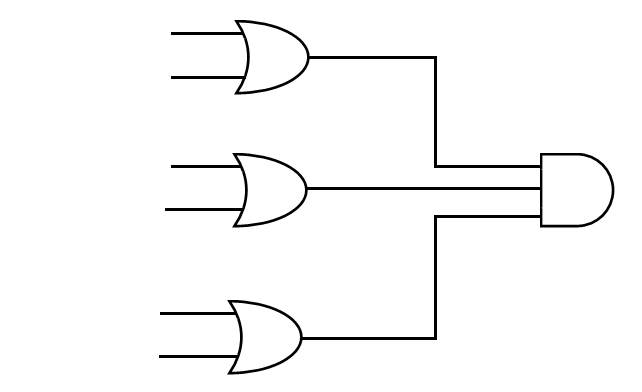A.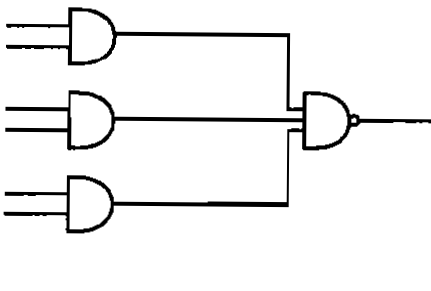B.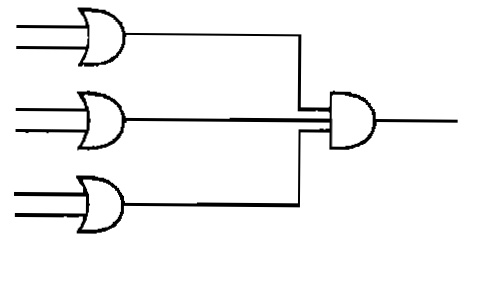C.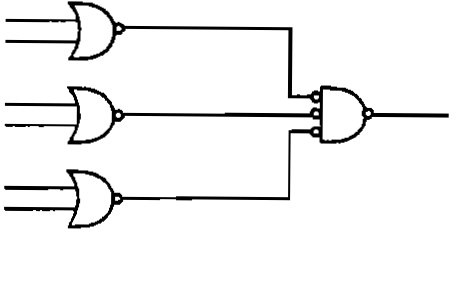D.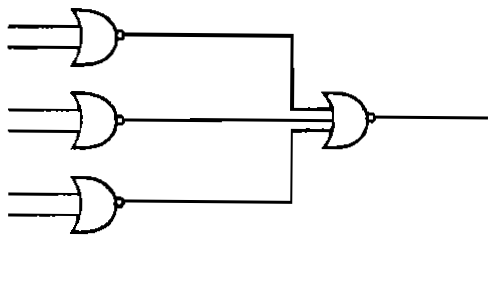Answer Report Discuss Option: B Explanation : Click on Discuss to view users comments. wdw said: (4:20pm on Monday 6th January 2014) Please Explain how ? Write your comments here:
48:

The black box in the following figure consists of a minimum complexity circuit that uses only AND,OR and NOT gates. The function f (x,y,z) = 1 whenever x , y are different and 0 otherwise. In addition the 3 inputs x,y,z are never all the same value. Which of the following equation lead to the correct design for the minimum complexity circuit?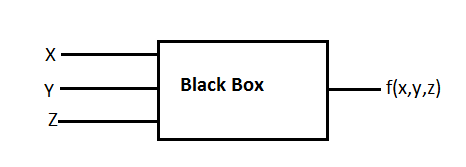A. x'y + xy' B. x + y'z C. x'y'z' + xy'z D. xy + y'z + z' Answer Report Discuss Option: A Explanation : Click on Discuss to view users comments. rehan said: (6:41pm on Saturday 28th February 2015) A part not contain the value of Z so how it full fill the function ????????? shrarik_zack said: (11:00am on Tuesday 29th May 2018) i think its driven with boolean function Write your comments here:
49:

If A ⊕ B = C, then

 A. A ⊕ C = B B. B ⊕ C = A C. A ⊕ B ⊕ C = 0 D. Both (a) & (b) Answer Report Discuss Option: D Explanation : Mathematically, XOR is both associative and commutative ie.   If C = A  XOR  B then B = C  XOR  A or  B = A  XOR  C and   and A = B  XOR C   A = C  XOR  B Click on Discuss to view users comments. bikash said: (4:05am on Wednesday 4th September 2013) Option C is also correct bikash said: (4:05am on Wednesday 4th September 2013) Option C is also correct Write your comments here:
50:

To make the following circuit a tautology ? marked box should beA. OR gate B. AND gate C. NAND gate D. EX-OR GATE Answer Report Discuss Option: C Explanation : The output f = (x+x')+(y+y'). Starting derivation using 'f'. -->(x+x')+(y+y') -->(x+y)+(x'+y') -->(Already a known Input)+(x'+y') So, the unknown input is (x'+y'). This can be made by :- x and y fed into a NOT gate and then AND gate to become (x'+y'). So the answer is NAND gate. Click on Discuss to view users comments. swetha k said: (8:02pm on Monday 5th October 2015) it should be XOR isn't it?? Sachin Raj G.S said: (10:23pm on Wednesday 4th April 2018) C is the exact answer for this question. 1st input is x y2nd input will be x' y'(nand gate)Next there is a and gate, so output should become,f=(x y).(x' y') =xx' xy' yx' yy' =1 xy' yx' 1 =1(because 1 a=1) Write your comments here:X